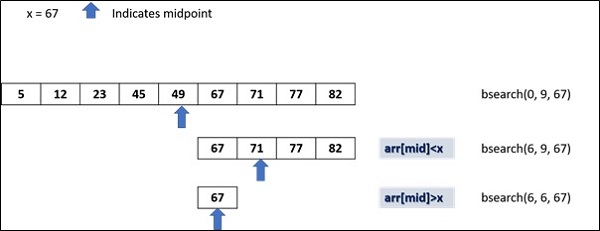# Python - Recursion

A function that calls itself is called a recursive function. This method is used when a certain problem is defined in terms of itself. Although this involves iteration, using iterative approach to solve such problem can be tedious. Recursive approach provides a very concise solution to seemingly complex problem.

The most popular example of recursion is calculation of factorial. Mathematically factorial is defined as −

```n! = n Ã— (n-1)!
```

It can be seen that we use factorial itself to define factorial. Hence this is a fit case to write a recursive function. Let us expand above definition for calculation of factorial value of 5.

```5! = 5 Ã— 4!
5 Ã— 4 Ã— 3!
5 Ã— 4 Ã— 3 Ã— 2!
5 Ã— 4 Ã— 3 Ã— 2 Ã— 1!
5 Ã— 4 Ã— 3 Ã— 2 Ã— 1
= 120
```

While we can perform this calculation using a loop, its recursive function involves successively calling it by decrementing the number till it reaches 1.

### Example 1

The following example shows hows you can use a recursive function to calculate factorial −

```def factorial(n):

if n == 1:
print (n)
return 1
else:
print (n,'*', end=' ')
return n * factorial(n-1)

print ('factorial of 5=', factorial(5))
```

It will produce the following output

```5 * 4 * 3 * 2 * 1
factorial of 5= 120
```

Let us have a look at another example to understand how recursion works. The problem at hand is to check whether a given number is present in a list.

While we can perform a sequential search for a certain number in the list using a for loop and comparing each number, the sequential search is not efficient especially if the list is too large. The binary search algorithm that checks if the index 'high' is greater than index 'low. Based on value present at 'mid' variable, the function is called again to search for the element.

We have a list of numbers, arranged in ascending order. The we find the midpoint of the list and restrict the checking to either left or right of midpoint depending on whether the desired number is less than or greater than the number at midpoint.

The following diagram shows how binary search works −### Example 2

The following code implements the recursive binary searching technique −

```def bsearch(my_list, low, high, elem):
if high >= low:
mid = (high + low) // 2
if my_list[mid] == elem:
return mid
elif my_list[mid] > elem:
return bsearch(my_list, low, mid - 1, elem)
else:
return bsearch(my_list, mid + 1, high, elem)
else:
return -1

my_list = [5,12,23, 45, 49, 67, 71, 77, 82]
num = 67
print("The list is")
print(my_list)
print ("Check for number:", num)
my_result = bsearch(my_list,0,len(my_list)-1,num)

if my_result != -1:
print("Element found at index ", str(my_result))
else:
```

It will produce the following output

```The list is
[5, 12, 23, 45, 49, 67, 71, 77, 82]
Check for number: 67
Element found at index 5
```

You can check the output for different numbers in the list, as well as not in the list.